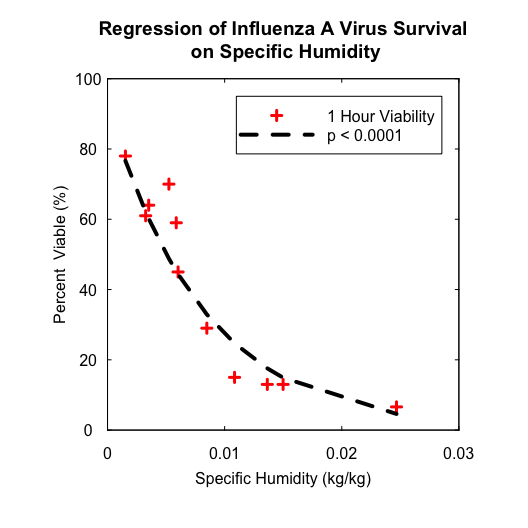# Regression of Influenza A Virus Survival on Specific Humidity

#### 2018-06-11

Harper (1961) tested airborne virus particles of influenza A for viable survival in the dark at controlled temperature and relative humidity for up to 23 hour after spraying. The 1-h influenza A virus survival data are extracted from the paper and now are stored in humidity pacakge with a dataset name of ivs. In this vignette, the dataset ivs is used to explore the relationship between influenza A virus survival and specific humidity. As specific humidity isn’t provided, we first need to apply functions from humidity package to calculate specific humidity from temperature and relative humidity.

# load humidity package
library(humidity)

# display built-in 1-h influenza virus surival data
head(ivs)
##       T   RH PVV
## 1  7.50 24.0  78
## 2  7.50 51.0  61
## 3  7.50 82.0  70
## 4 22.25 21.0  64
## 5 22.25 35.0  59
## 6 22.25 50.5  29

As the temperature T is recorded in degree Celsius (°C), we first apply C2K function to convert the temperature into Kelvin (K) before our following calculation. We then call SVP and WVP functions to calculate saturation vapor pressure $$e_s$$ (hPa) and partial water vapor pressure $$e$$ (Pa) at temperature $$T$$, respectively. Finally by calling AH, SH, and MR functions, we can calculate humidity measures of interest, such as absolute humidity $$\rho_w$$ (kg/m^3), specific humidity $$q$$ (kg/kg), and mixing ratio $$\omega$$ (kg/kg).

Note that SVP function provides two formulas (either Clausius-Clapeyron equation or Murray equation) for calculating saturation vapor pressure. Both results are the same and the default formula is Clausius-Clapeyron, which is consistent with Shaman and Kohn (2009). Furthermore, Harper (1961) didn’t give any information on the atmospheric pressure condition under which his experiments were conducted. We just assume that he performed the experiments under standard atmospheric pressure and the default value of p = 101325 Pa is used in SH function when calculating specific humidity.

library(tidyverse)
ivs <- ivs %>%
mutate(Tk = C2K(T), # tempature in Kelvin
Es = SVP(Tk), # saturation vapor pressure in hPa
E = WVP2(RH, Es), # partial water vapor pressure in Pa
rho = AH(E, Tk), # absolute humidity in kg/m^3
q = SH(E), # specific humidity in kg/kg
omega = MR(q), # mixing ratio in kg/kg
)
# display calculation results
head(ivs)
##       T   RH PVV     Tk       Es         E         rho           q
## 1  7.50 24.0  78 280.65 10.38008  249.1219 0.001923341 0.001530702
## 2  7.50 51.0  61 280.65 10.38008  529.3840 0.004087100 0.003256149
## 3  7.50 82.0  70 280.65 10.38008  851.1665 0.006571416 0.005241681
## 4 22.25 21.0  64 295.40 27.21156  571.4428 0.004191522 0.003515398
## 5 22.25 35.0  59 295.40 27.21156  952.4047 0.006985870 0.005867353
## 6 22.25 50.5  29 295.40 27.21156 1374.1839 0.010079613 0.008479141
##         omega
## 1 0.001533048
## 2 0.003266786
## 3 0.005269301
## 4 0.003527799
## 5 0.005901982
## 6 0.008551651

Hinted by Shaman and Kohn (2009) and Shaman et al. (2010), log-linear regression model is applied to describe the relationship between influenza A virus survival and specific humidity.

# log-linear regression of 1-h infuenza A virus survival on specific humididty
loglm <- lm(log(PVV) ~ q, data = ivs)
summary(loglm)
##
## Call:
## lm(formula = log(PVV) ~ q, data = ivs)
##
## Residuals:
##      Min       1Q   Median       3Q      Max
## -0.49834 -0.13209  0.01414  0.16364  0.36192
##
## Coefficients:
##              Estimate Std. Error t value Pr(>|t|)
## (Intercept)    4.5249     0.1445  31.323 1.69e-10 ***
## q           -121.5782    13.1247  -9.263 6.74e-06 ***
## ---
## Signif. codes:  0 '***' 0.001 '**' 0.01 '*' 0.05 '.' 0.1 ' ' 1
##
## Residual standard error: 0.281 on 9 degrees of freedom
## Multiple R-squared:  0.9051, Adjusted R-squared:  0.8945
## F-statistic: 85.81 on 1 and 9 DF,  p-value: 6.74e-06

The regression coefficient of specific humidity $$q$$ is significant with a $$p$$-value less than 0.0001, indicating that log-linear model can well depict the association of influenza virus survival with specific humidity. But the coefficient estimate for specific humidity $$q$$ is -121.5782, which is too far from the value of $$a = -180$$ estimated by Shaman et al. (2010).

The following codes draw the scatter plot of 1-h influenza A virus survival and specific humidity, with regression curve overlapped.

# get fitted value to plot regression curve
ivs$fit.val <- exp(predict(loglm)) ivs <- ivs[with(ivs, order(q)), ] # plot percentage viable virus vs. specific humidity par(pty = "s") plot(x = ivs$q, y = ivs$PVV, col = "red", pch = 3, lwd = 3, xaxt = "n", yaxt = "n", xlim = c(0, 0.03), ylim = c(0, 100), xaxs = "i", yaxs = "i", xlab = "", ylab = "", main = "Regression of Influenza A Virus Survival\n on Specific Humidity") title(xlab = "Specific Humidity (kg/kg)", ylab = "Percent Viable (%)", mgp = c(2, 1, 0)) # plot regression curve lines(x = ivs$q, y = ivs\$fit.val, lty = "dashed", lwd = 4)
axis(side = 1, at = seq(0, 0.03, by = 0.01), labels = c("0", "0.01", "0.02", "0.03"),
tck = 0.01, padj = -0.5)
axis(side = 2, at = seq(0, 100, by = 20), tck = 0.01, las = 2, hadj = 0.5)
axis(side = 3, at = seq(0, 0.03, by = 0.01), labels = FALSE, tck = 0.01)
axis(side = 4, at = seq(0, 100, by = 20), labels = FALSE, tck = 0.01)
legend(0.011, 95, legend = c("1 Hour Viability", "p < 0.0001"), pch = c(3, NA),
col = c("red", "black"), lty = c(NA, "dashed"), lwd = c(3, 4), seg.len = 5)The codes also reproduce the Figure 1.(B) of Shaman et al. (2010).